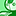# QlikView App Dev

Discussion Board for collaboration related to QlikView App Development.

Announcements
Join this live chat April 6, 10AM EST - QlikView to Qlik Sense REGISTER
cancel
Showing results for
Did you mean:Not applicable

## How to only show certain data in a straight table

Hey,

I have a straight table with the following two dimensions and four expressions.

Dimensions:

CustomerNumber
CustomerName

Expressions:

Turnover =sum(Amount_Turnover)
Cost =Sum(Amount_Royalty)+Sum(Amount_Sales)
EBT =Sum(Amount_Turnover)-(Sum(Amount_Royalty)+Sum(Amount_Sales)
EBT degree (In percent)
=(Sum(Amount_Turnover)-(Sum(Amount_Royalty)+Sum(Amount_Sales)))/(Sum(Amount_Turnover)

I need my table to show only the customers which Amount_Turnover is more than zero and which EBT degree is 25% or less.

I need to do it without editing the script, so is it somehow possible to do this using the Chart Properties?

Kind regards,

Peter

2 RepliesSpecialist II

yes possible...

look at dimension limits tab of chart properties..

or share a sample qvw...Contributor

Hi,